## Example Questions

### Example Question #163 : Solid Geometry

What is the surface area of a cone with a radius of 4 and a height of 3?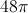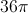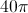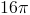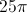Explanation:

Here we simply need to remember the formula for the surface area of a cone and plug in our values for the radius and height.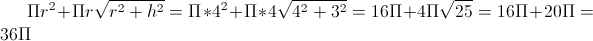### Example Question #162 : Solid Geometry

The lateral area is twice as big as the base area of a cone.  If the height of the cone is 9, what is the entire surface area (base area plus lateral area)?

81π

90π

27π

54π

81π

Explanation:

Lateral Area = LA = π(r)(l) where r = radius of the base and l = slant height

LA = 2B

π(r)(l) = 2π(r2)

rl = 2r2

l = 2r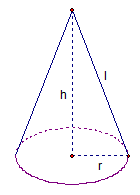From the diagram, we can see that r2 + h2 = l2.  Since h = 9 and l = 2r, some substitution yields

r2 + 92 = (2r)2

r2 + 81 = 4r2

81 = 3r2

27 = r2

B = π(r2) = 27π

LA = 2B = 2(27π) = 54π

SA = B + LA = 81π

### Example Question #861 : Geometry

A right cone has a radius of 4R and a height of 3R. What is the ratio of the total surface area of the cone to the surface area of just the base?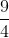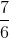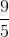Explanation:

We need to find total surface area of the cone and the area of the base.

The area of the base of a cone is equal to the area of a circle. The formula for the area of a circle is given below:, where r is the length of the radius.

In the case of this cone, the radius is equal to 4R, so we must replace r with 4R.To find the total area of the cone, we need the area of the base and the lateral surface area of the cone. The lateral surface area (LA) of a cone is given by the following formula: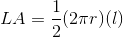, where r is the radius and l is the slant height.

We know that r = 4R. What we need now is the slant height, which is the distance from the edge of the base of the cone to the tip.

In order to find the slant height, we need to construct a right triangle with the legs equal to the height and the radius of the cone. The slant height will be the hypotenuse of this triangle. We can use the Pythagorean Theorem to find an expression for l. According to the Pythagorean Theorem, the sum of the squares of the legs (which are 4R and 3R in this case) is equal to the square of the hypotenuse (which is the slant height). According to the Pythagorean Theorem, we can write the following equation: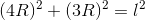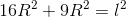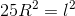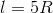Let's go back to the formula for the lateral surface area (LA).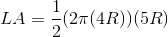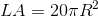To find the total surface area (TA), we must add the lateral area and the area of the base.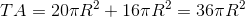The problem requires us to find the ratio of the total surface area to the area of the base. This means we must find the following ratio: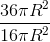We can cancel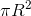, which leaves us with 36/16.

Simplifying 36/16 gives 9/4.

### Example Question #2 : Cones

You are given a right circular cone with height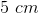. The radius is twice the length of the height. What is the volume?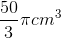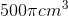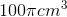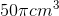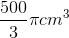Explanation:

You are given a right circular cone with height 5. The radius is twice the length of the height. What is the volume?

Height = 5cm. The radius is twice the height.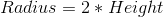, so the radius is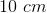.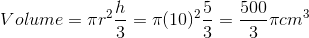### Example Question #3 : Cones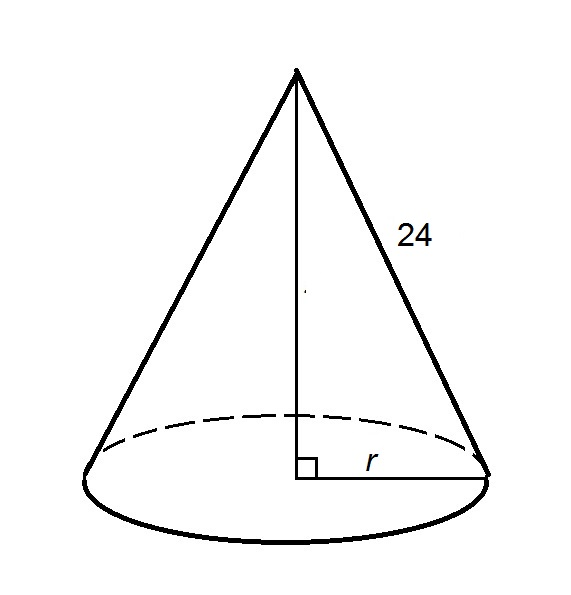In terms of, express the surface areaof the above right circular cone.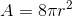None of the other choices gives the correct response.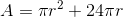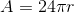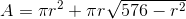Explanation:

The surface areaof a right circular cone, given its slant heightand the radiusof its base, can be found using the formula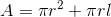The slant height is shown to be 24, so setting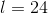and substituting: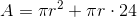### Example Question #4 : Cones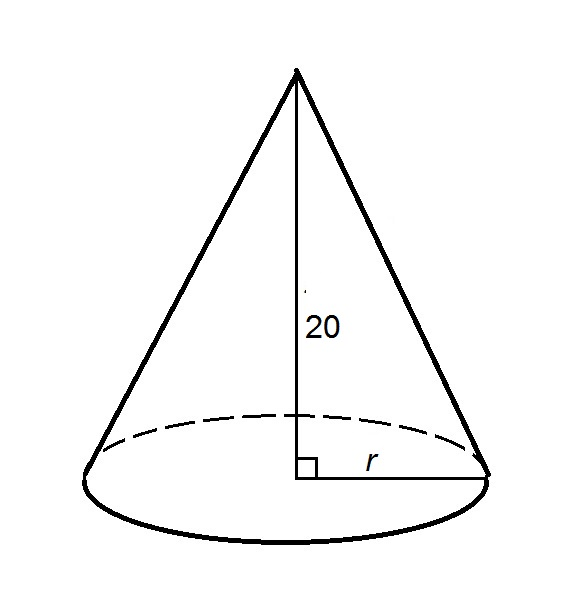In terms of, express the surface areaof the provided right circular cone.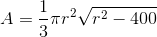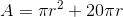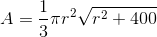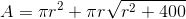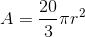Explanation:

The surface areaof a right circular cone, given its slant heightand the radiusof its base, can be found using the formulaThe heightis shown in the diagram to be 20. By the Pythagorean Theorem,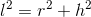Setting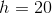and solving for: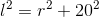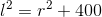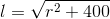Substituting in the surface area formula: